Next: Bibliography Up: 12. Power Series Previous: 12.8 Proof of the   Index

# 12.9 Some XVIII-th Century Calculations

The following proofs that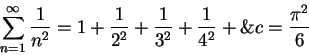and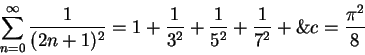use XVIII-th century standards or rigor. You should decide what parts are justified. I denote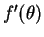by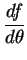below. By the geometric series formula,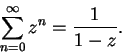Ifwhere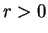,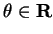, then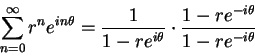so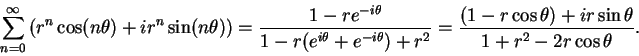By equating the real and imaginary parts, we get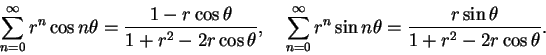For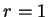, this yields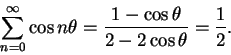Thus,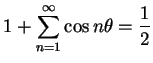, so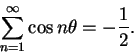Hence,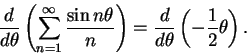Since two antiderivatives of a function differ by a constantfor some constant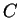. When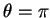, we get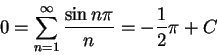so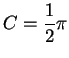and thus(12.79)

For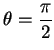, this gives us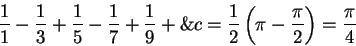(which is the Gregory-Leibniz-Madhava formula). We can rewrite (12.79) as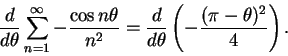Again, since two antiderivatives of a function differ by a constant, there is a constantsuch that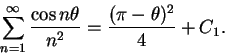For, this says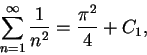and for, this says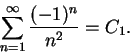Subtract the second equation from the first to get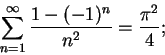i.e.,and thus(12.80)

Let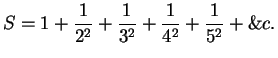Subtract (12.80) from this to get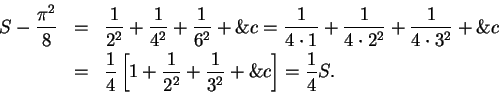Hence,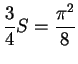, and then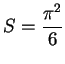.An argument similar to the following was given by Jacob Bernoulli in 1689 [31, p 443]. Let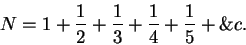Then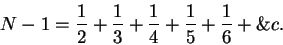Subtract the second series from the first to get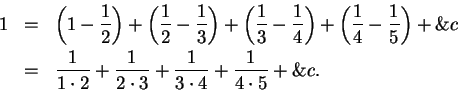Therefore,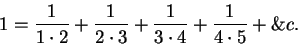12.81   Exercise.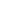a) Explain why Bernoulli's argument is not valid.
b) Give a valid argument proving that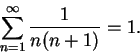12.82   Note. The notationwas introduced by William Jones in 1706 to represent the ratio of the circumference to the diameter of a circle[15, vol2, p9]. Both Maple and Mathematica designateby Pi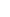.

The notation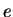was introduced by Euler in 1727 or 1728 to denote the base of natural logarithms[15, vol 2, p 13]. In Mathematicais denoted by E. In the current version of Maple there is no special name for; it is denoted by exp(1).Next: Bibliography Up: 12. Power Series Previous: 12.8 Proof of the   Index